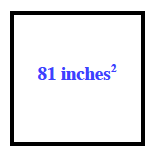### Home > CC1MN > Chapter 10 > Lesson 10.1.1 > Problem10-9

10-9.

A square has an area of $81$ square inches. What is its perimeter?

Remember that perimeter is the distance around a shape. Also, a square has four sides which are equal in length.

Here is a sketch of a square with an area of $81$ square inches. What number multiplied by itself equals $81$? That number is the length of one side of the square.